Introduction to the Overall Concordance Correlation Coefficient

5/2/03

Oral Exam Presentation

Can a new technique or instrument reproduce the results of a "gold-standard"?

Examples:

• Mercury blood pressure cuff vs. electronic digital cuff

• Machines for measuring bilirubin in blood

• Magnetic resonance imagery vs. intra-arterial angiogram

How would a graduate student explore the question? I surveyed 18 graduate students and the responses were:

Paired t-test: 55.56%

Linear regression: 22.22%

Only graphs: 11.11%

Other: 11.11%

By a paired t-test, testing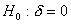by the test statistic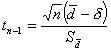By linear regression, we want slope = 1, and intercept = 0, by the test statisticsBy the Pearson correlation coefficient,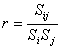, testing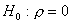by the test statistic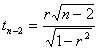Problems:

Paired t-test tests only whether the means are equal

Least squares regression analysis is typically misused by regressing one measurement on the other and declaring them equivalent if and only if the confidence interval for the regression coefficientincludes 1

The Pearson correlation coefficient only measures linear correlation, but fails to detect departure from theline

The Concordance Correlation Coefficient (CCC) addresses these issues

Consider pairs of samples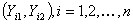It is natural to consider the expected squares difference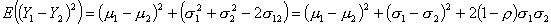To scale it between -1 and 1

CCC =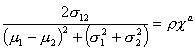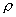is a measure of precision (deviation from best-fit line)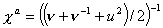is a measure of accuracy (deviation fromline)is a scale shift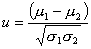is a location shift relative to the scale

Characteristics

•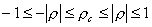•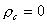if and only if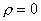•if and only if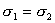and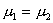•if and only if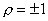,andThe CCC

• "evaluates the degree to which pairs fall on the 45 degree line through the origin"
• has natural, familiar interpretations
• is a measure of precision and accuracy

The estimate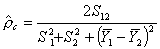Inference

•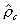is a consistent estimator for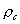and has asymptotic normality (for bivariate normal data)
• can improve the normal approximation by using the inverse hyperbolic tangent transformation: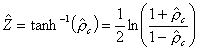• Z transformation is able to provide a more realistic asymmetric interval (Serfling, 1980)
• Z is robust against non-normality for samples from uniform and Poisson distributions, even with a sample size of 10 (Lin, simulations, 1989)

Overall Concordance Correlation Coefficient

• Need to assess agreement amongobservers/methods
• Natural to use, the inter-observer variability, whereOCCC =, whereOCCC can be interpreted as the weighted average of all pairwise CCC's

We can rewrite the OCCC as a function of means, variances, and covariances as:And we can also rewrite as product of precision and accuracy:Simulation

• U-statistics
• GEE
• Bootstrap

Table 1 (p. 1023)

 TrueTrueN Mean SD Mean SE .5 .469 100 .4636 .0502 .0512 50 .4582 .0735 .0719 25 .4486 .1016 .1012 .7 .656 100 .6489 .0404 .0401 50 .6455 .0585 .0558 25 .6355 .0887 .0866 .9 .844 100 .8409 .0224 .0224 50 .8372 .0336 .0323 25 .8337 .0451 .04505

Extending the OCCC

• Alternatives to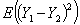?